#Function Repository Resource:

# WolframModelPlot

Generate a visual display of a hypergraph

Contributed by: Wolfram Research
 ResourceFunction["WolframModelPlot"][g] generates a plot of the hypergraph g. ResourceFunction["WolframModelPlot"][{g1,g2,…}] generates a list of plots of the hypergraphs gi. ResourceFunction["WolframModelPlot"][data,"type"] generates plots assuming hypergraphs of the specified type.

## Details and Options

ResourceFunction["WolframModelPlot"] attempts to place vertices to give a well-laid-out version of the hypergraph.
In ResourceFunction["WolframModelPlot"][g], g should be a list of hyperedges: {{n11,n12,},{n21,},}.
Unary edges are supported and displayed as circles around the vertices.
In ResourceFunction["WolframModelPlot"][data,"type"], possible types of hypergraphs to assume include:
 "Ordered" every hyperedge is interpreted as a sequence of directed edges of adjacent vertices "Cyclic" the same as "Ordered", with an addition of a directed edge from the last vertex to the first
WolframModelPlot has the same options as Graphics, with following additions:
 "ArrowheadLength" Automatic the length of arrowheads "EdgePolygonStyle" Automatic style for hyperedge polygons "HyperedgeRendering" "Polygons" how to render hyperedges in the hypergraph "MaxImageSize" Automatic upper limit for the image size EdgeStyle Automatic style for edges GraphHighlight {} vertices and hyperedges to highlight GraphHighlightStyle Red highlight color PlotStyle Automatic overall style VertexCoordinateRules {} coordinates specified for particular vertices VertexLabels None labels for vertices VertexSize 0.06 the size of vertices VertexStyle Automatic style for vertices
Possible settings for "HyperedgeRendering" include:
 "Subgraphs" render hyperedges as ordinary graphs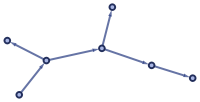"Polygons" render hyperedges as polygons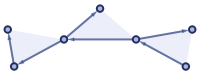Install the SetReplace paclet to get better performance and bleeding-edge functionality.
See the SetReplace README for a more detailed documentation.

## Examples

### Basic Examples (3)

Plot a hypergraph:

 In:=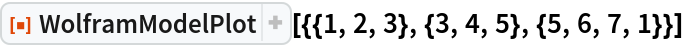Out=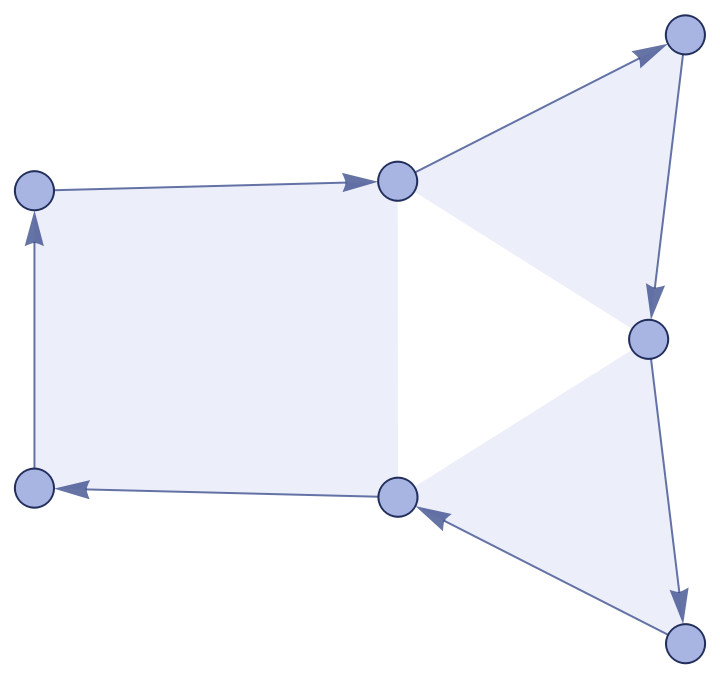Plot multiple hypergraphs:

 In:=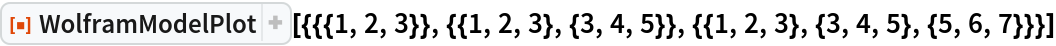Out=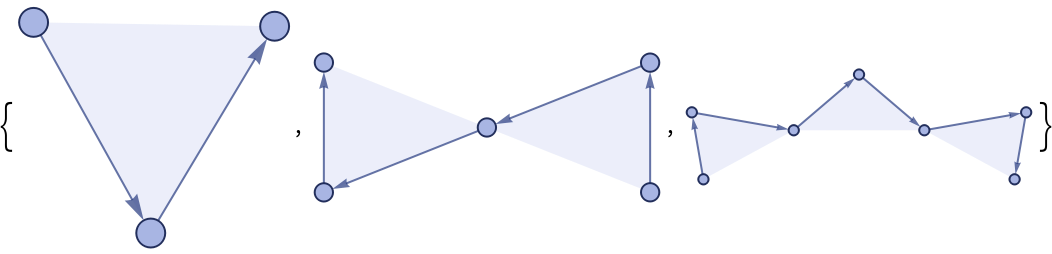Plot a cyclic hypergraph:

 In:=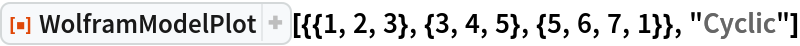Out=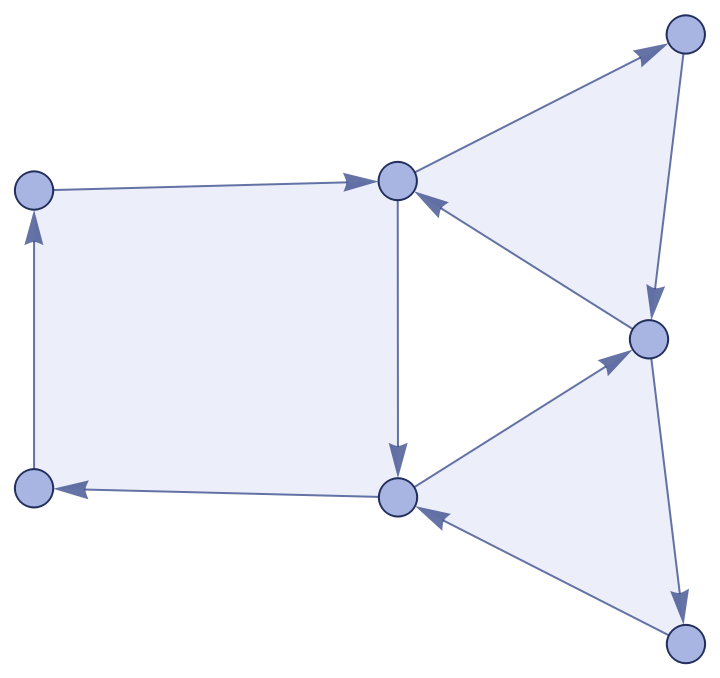### Scope (4)

Unary edges are shown as circles around the vertices:

 In:=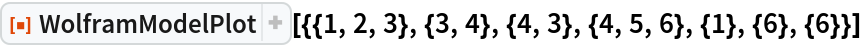Out=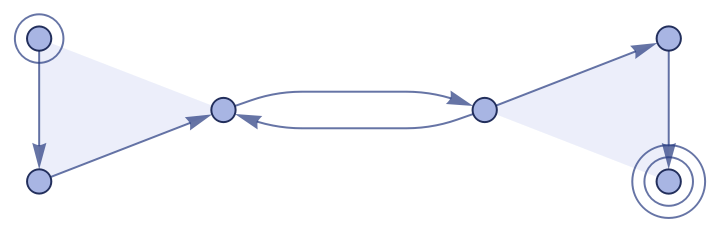Self-loops are shown as convex polygons around the appropriate number of circular arrows:

 In:=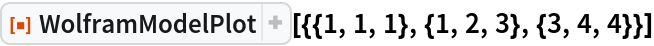Out=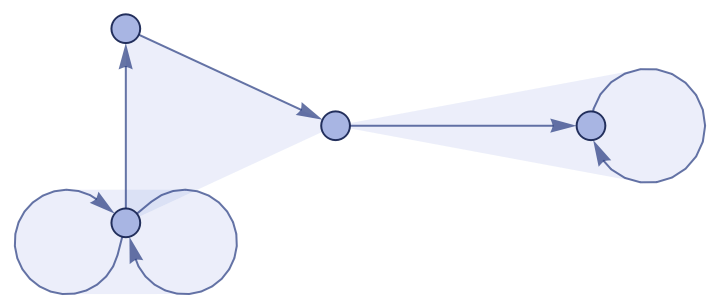Note the difference between a hyper-self-loop and two binary edges pointing in opposite directions:

 In:=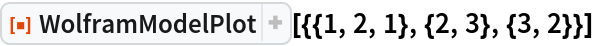Out=Multiedges are shown as multiple arrows and polygons:

 In:=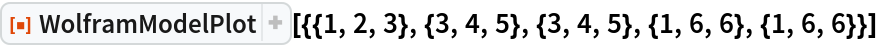Out=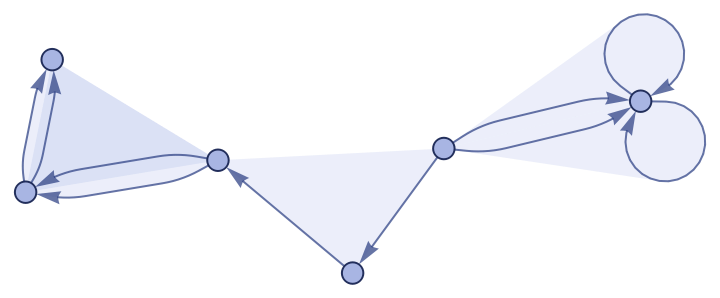### Options (17)

#### GraphHighlight (1)

Highlight some vertices and edges:

 In:=Out=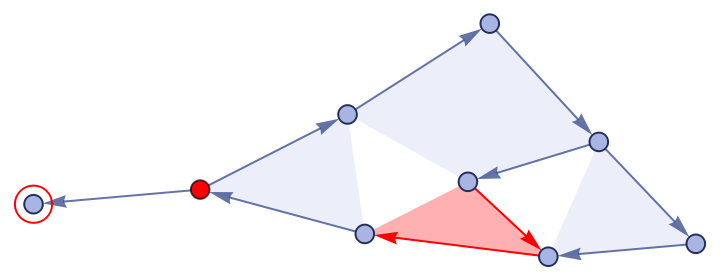#### GraphHighlightStyle (1)

Change the color of the highlight:

 In:=Out=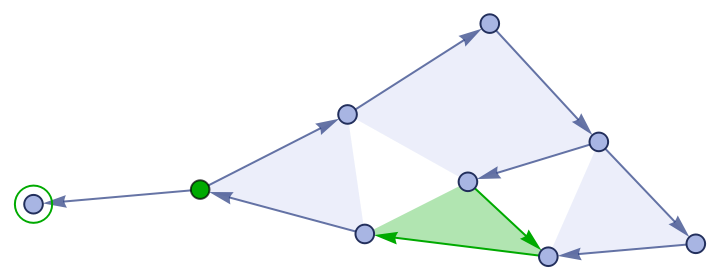#### HyperedgeRendering (1)

Unroll each hypergraph to a sequence of binary edges:

 In:=Out=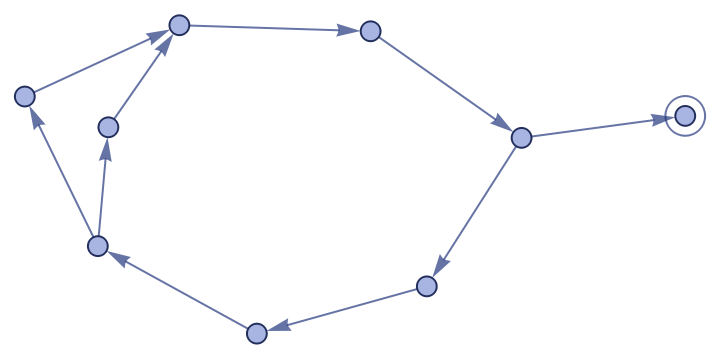#### VertexCoordinateRules (1)

Specify custom coordinates for the vertices:

 In:=Out=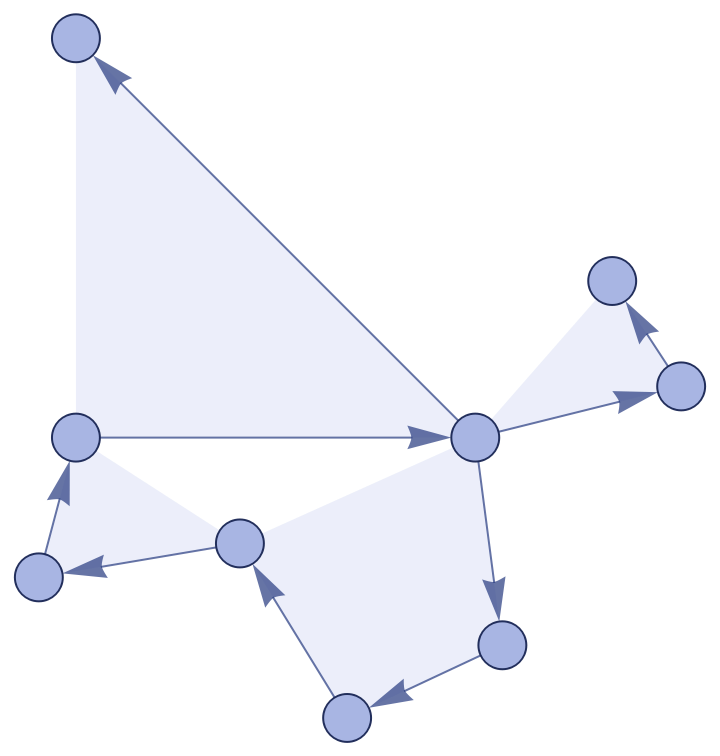#### VertexLabels (1)

Display the labels for vertices:

 In:=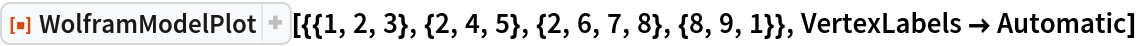Out=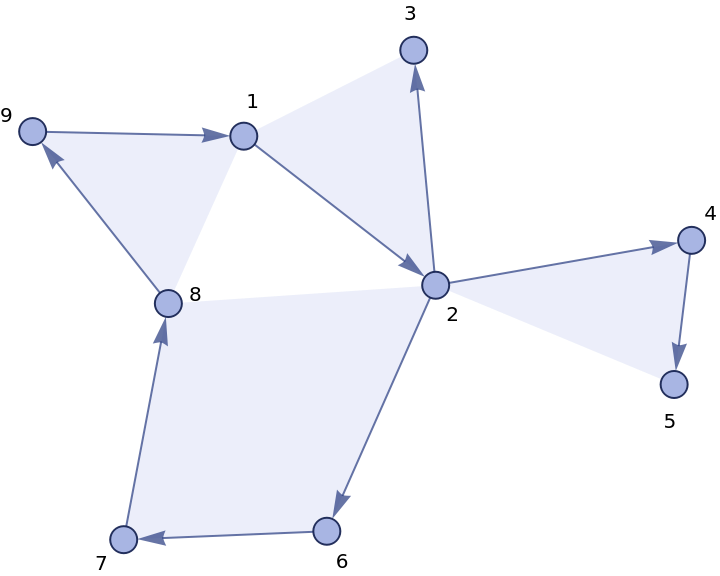#### VertexSize (1)

Make vertices appear larger:

 In:=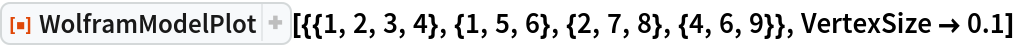Out=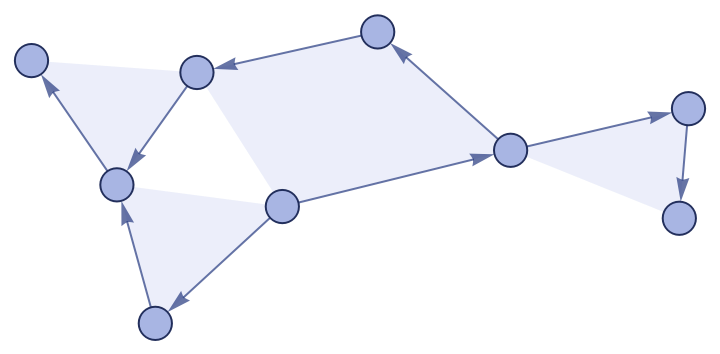In:=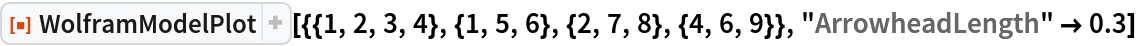Out=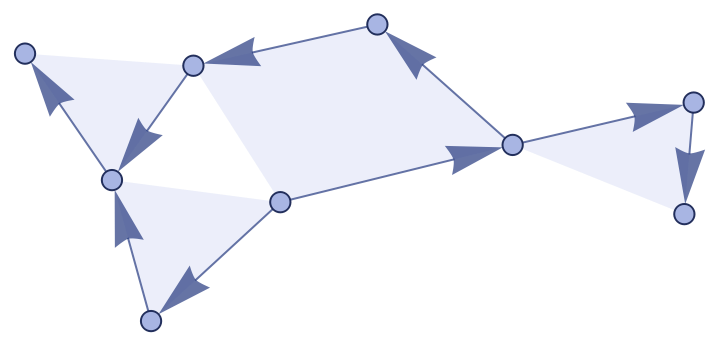#### MaxImageSize (1)

Reduce the maximum image size, but keep small hypergraphs small:

 In:=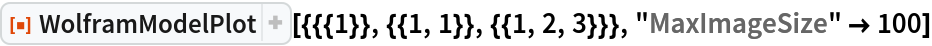Out=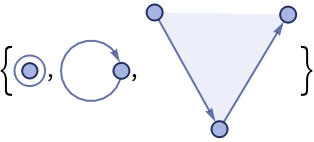#### PlotStyle (1)

Specify an overall style:

 In:=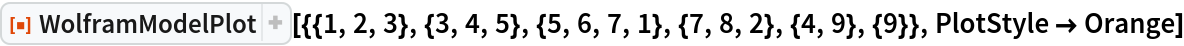Out=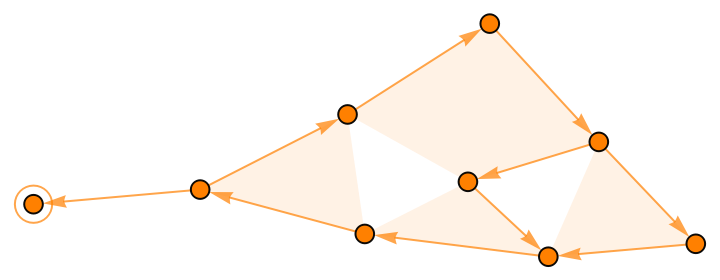#### VertexStyle (3)

Specify a style for vertices:

 In:=Out=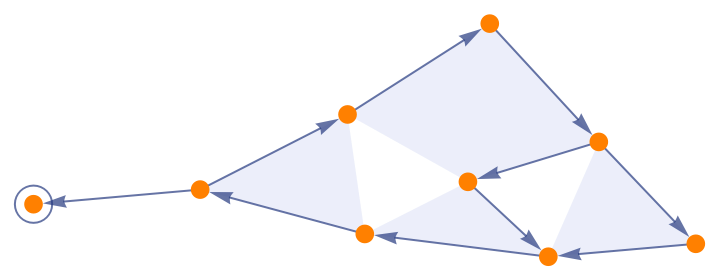Styles for each vertex can be given as a List:

 In:=Out=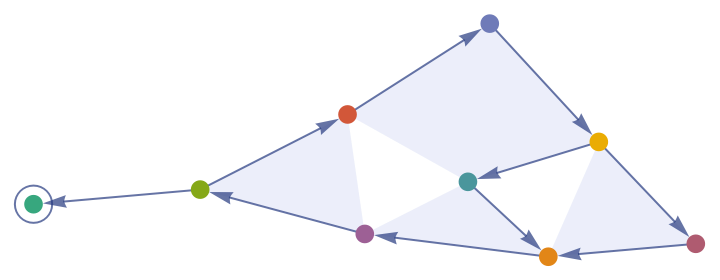Styles for different vertex patterns can be given as an Association:

 In:=Out=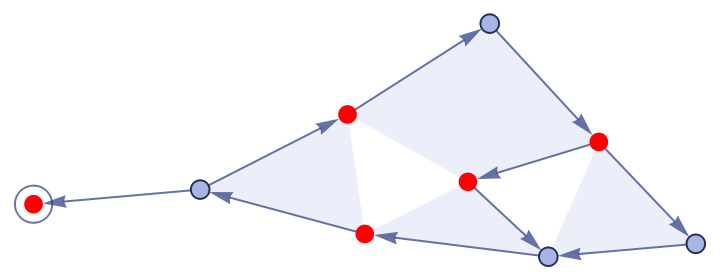#### EdgeStyle (3)

Specify a style for edges:

 In:=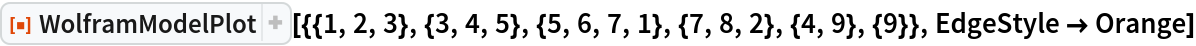Out=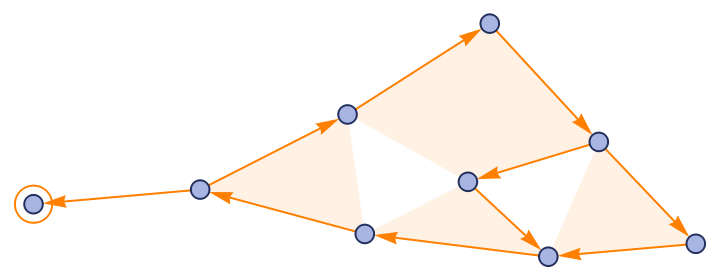Styles for each edge can be given as a List:

 In:=Out=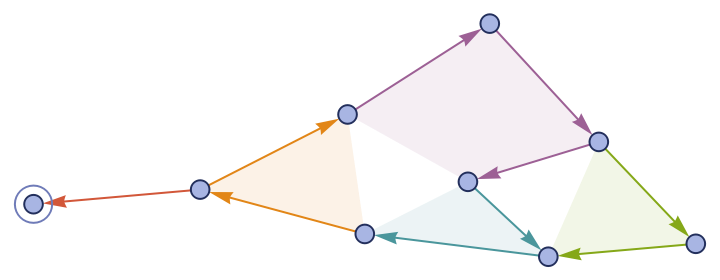Styles for different edge patterns can be given as an Association:

 In:=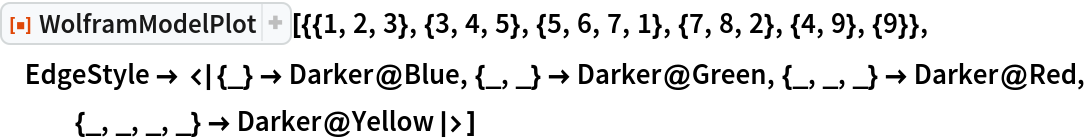Out=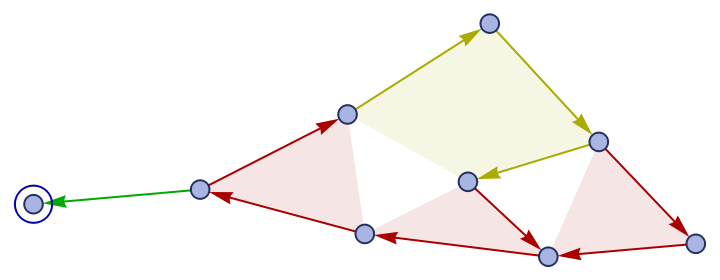#### EdgePolygonStyle (2)

Specify a style for edge polygons:

 In:=Out=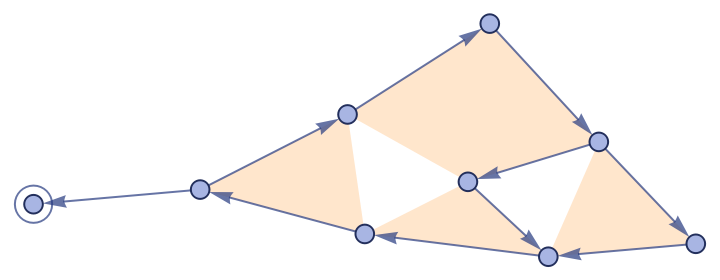This can be specified independently from the style for edge lines:

 In:=Out=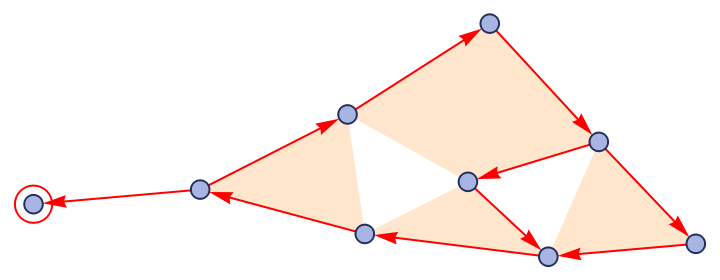### Properties and Relations (2)

WolframModelPlot is used in various properties of WolframModel:

 In:=Out=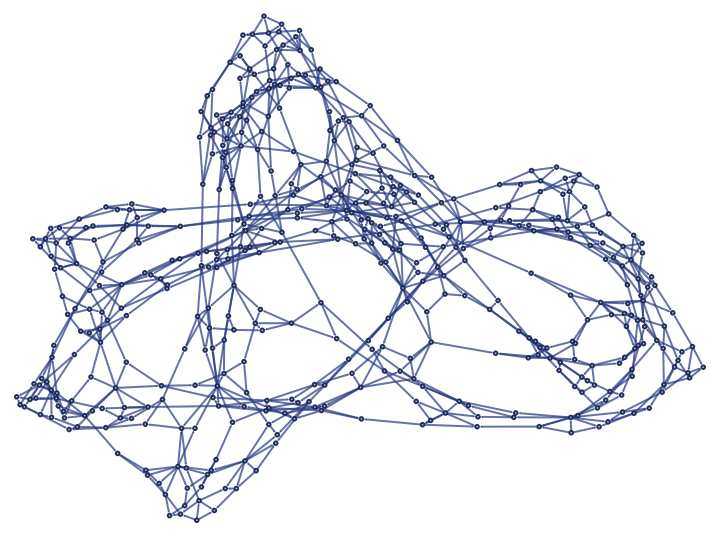It is also used in RulePlot:

 In:=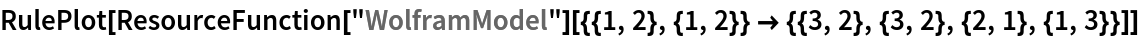Out=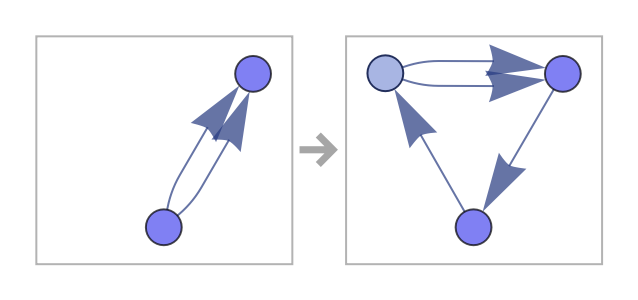### Possible Issues (2)

It may be hard to see the boundaries between hyperedges:

 In:=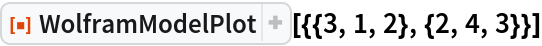Out=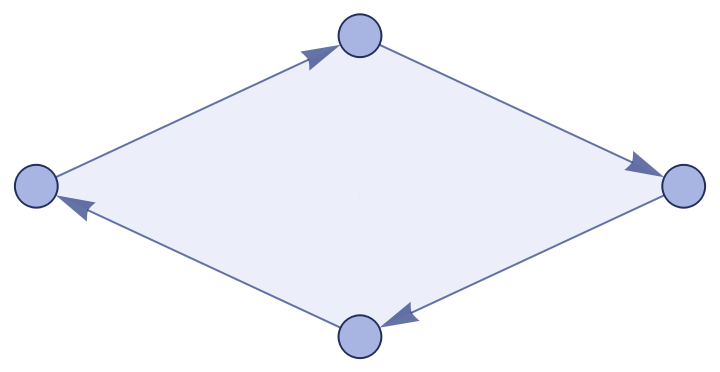Using different colors for each edge might help:

 In:=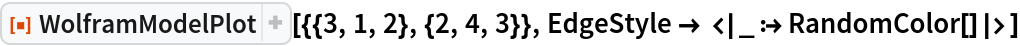Out=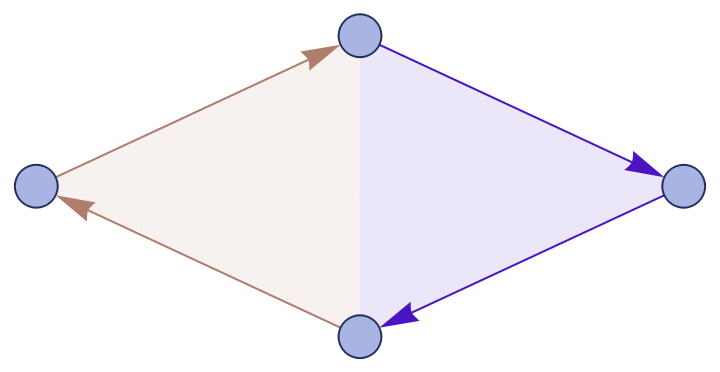Manually specifying coordinates might break the scaling, so vertices and arrowheads may appear too large relative to the distance between vertices:

 In:=Out=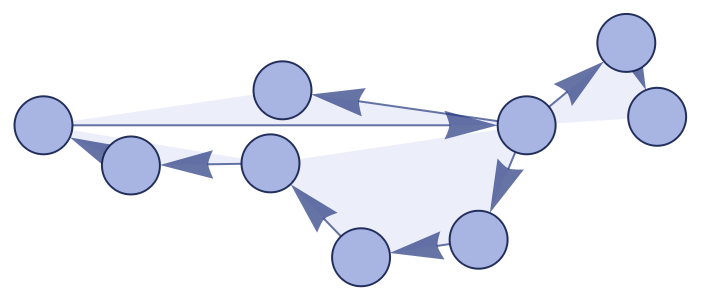Manually reduce the vertex/arrowhead sizes to get a better-looking image:

 In:=Out=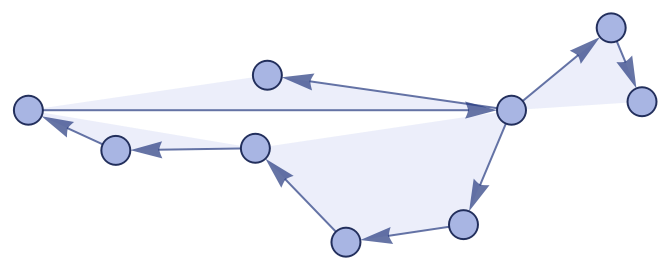### Neat Examples (3)

Make polygon art by getting rid of the vertices and arrowheads:

 In:=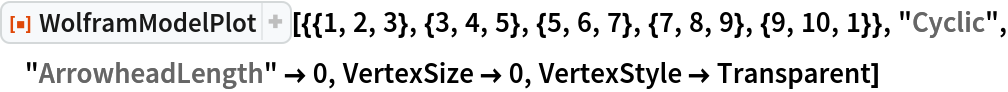Out=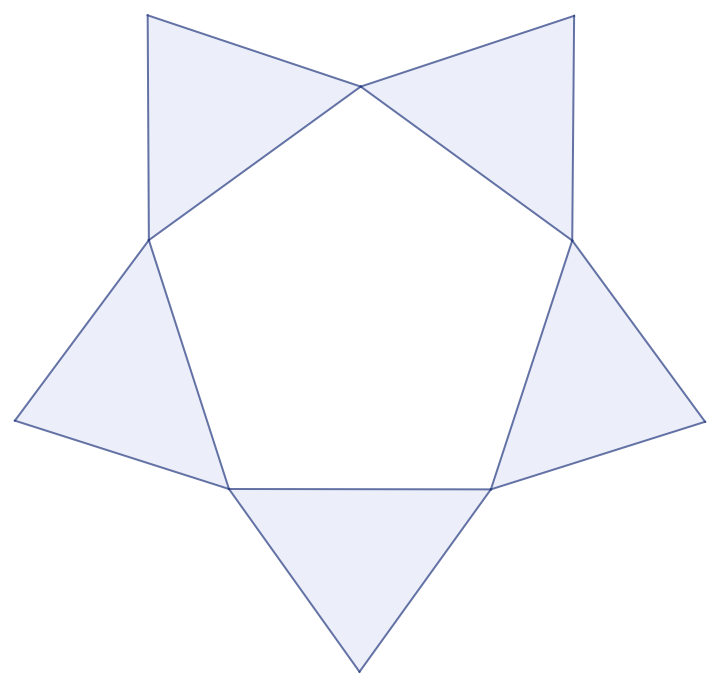Make an unordered hypergraph plot by specifying a custom edge style:

 In:=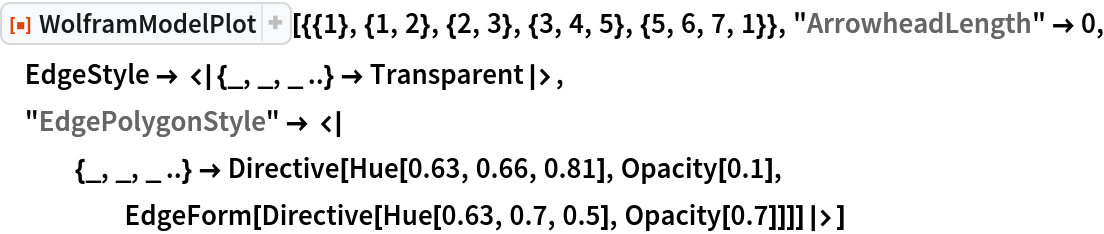Out=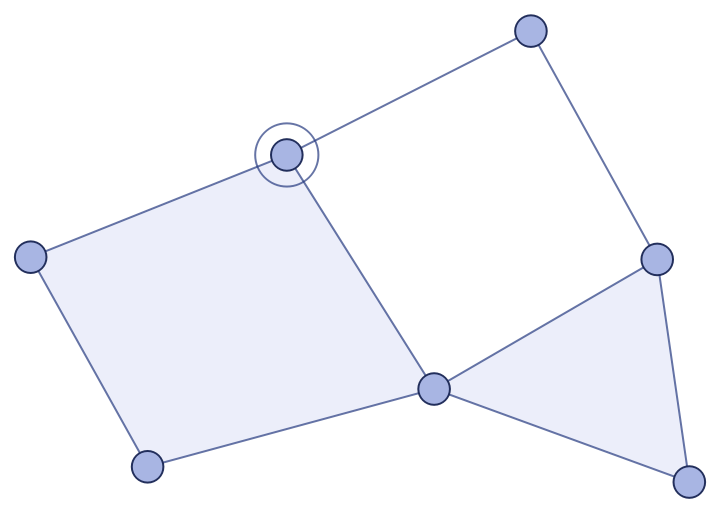Display binary and ternary edges in different colors:

 In:=Out=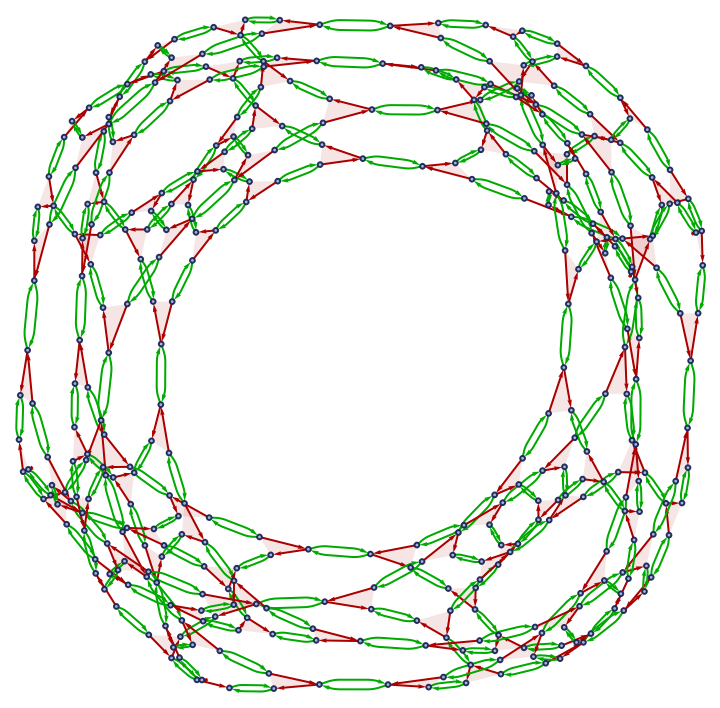## Version History

• 6.0.0 – 23 June 2020